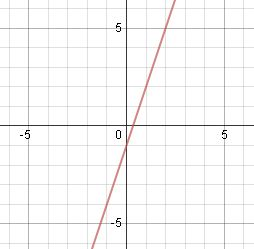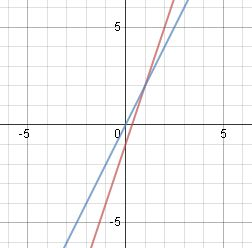# How to Solve a System of Equations by Graphing

An error occurred trying to load this video.

Try refreshing the page, or contact customer support.

Coming up next: How to Solve a System of Equations by Substitution

### You're on a roll. Keep up the good work!

Replay
Your next lesson will play in 10 seconds
• 0:05 A System of Equations
• 0:59 Graphing the First Line
• 2:06 Finding the Solution
• 3:18 Example
• 4:16 Lesson Summary

Want to watch this again later?

Timeline
Autoplay
Autoplay
Speed

#### Recommended Lessons and Courses for You

Lesson Transcript
Instructor: Yuanxin (Amy) Yang Alcocer

Amy has a master's degree in secondary education and has taught math at a public charter high school.

Watch this video lesson to learn how you can solve a system of equations by graphing. Learn when you have a solution and when you don't. Also learn how easy the solution is to spot.

## A System of Equations

When it comes to math, you won't always have the luxury of solving single equations. You might just also have to solve a system of equations. These are problems that include more than one equation. You can have two equations, three equations, or more. To solve these types of problems, you need to take into consideration all of the equations together. Your solution needs to fit them all. There are several methods that you can use to solve a system of equations.

In this lesson, you'll learn the graphing method. This method involves graphing the equations to solve. It is a visual way to solve your problem. Once you have graphed your equations, all you have to do is to look at the graph to find your solution. It is very easy to spot, as you will see.

Let's take a look at how you can solve a problem such as this:

y = 3x - 1

y = 2x

## Graphing the First Line

In order to use the graphing method, your equations must be in slope-intercept form so that you can easily graph them. Recall that the slope-intercept form is y = mx + b, where m is your slope and b is your y-intercept. The y-intercept tells you where the line crosses the y-axis and the m tells you what your slope is. After graphing the y-intercept, you use the slope to determine the angle of the line.

Looking at your equations, you see that they are already in slope-intercept form. If they weren't, then you would need to manipulate the equations to turn them into slope-intercept form. Since the equations are ready to use, you go ahead and graph the first line, y = 3x - 1. The y-intercept is -1 so you plot a point at (0, -1). Now, the slope is 3, so to find your next point from the y-intercept, you go up three and to the right one. This takes you to the point (1, 2). Now you connect these two dots and draw your line. You extend the line through your graph. You are done graphing the first line now.## Finding the Solution

To find your solution, you go on to graph the rest of your equations. You have just one more equation, so you go on to graph that one. The y-intercept is 0, so you plot a point at (0, 0). The slope is 2, so your next point is located two spaces up and one space to the right of the y-intercept. This next point is (1, 2). You connect these two dots and draw out your line.To unlock this lesson you must be a Study.com Member.

### Register to view this lesson

Are you a student or a teacher?

### Unlock Your Education

#### See for yourself why 30 million people use Study.com

##### Become a Study.com member and start learning now.
Back
What teachers are saying about Study.com

### Earning College Credit

Did you know… We have over 160 college courses that prepare you to earn credit by exam that is accepted by over 1,500 colleges and universities. You can test out of the first two years of college and save thousands off your degree. Anyone can earn credit-by-exam regardless of age or education level.